# The machine

The machine works 7 hours a day and produces 1 part in 5 minutes. How many parts will it produce in 1 hour? How many parts will it produce in 1 day?

Result

n1 =  12
n2 =  84

#### Solution:

$n_{ 1 } = \dfrac{ 60 }{ 5 } = 12$
$n_{ 2 } = n_{ 1 } \cdot \ 7 = 12 \cdot \ 7 = 84$

Leave us a comment of this math problem and its solution (i.e. if it is still somewhat unclear...):Be the first to comment!## Next similar math problems:

1. Third of an hour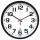How many minutes is a third of an hour? Do you know to determine a third of the lesson hour (45min)?
2. Pitcher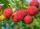Matthew picked up half-pitcher of raspberry in 45 minutes. Find how long three children filled the pitcher if each of them working at the same pace as Matthew.
3. Fishing boat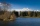The fishing boat caught 14 fish in one day. How many fish will catch 4 fishing boats in 8 days?
4. Bakers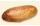Baker Martin baked 5 times more cakes than Dennis. How many cakes baked Dennis if Martin bake 25 cakes?
5. Multiples of int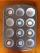How many multiples of 3 is between the numbers to 20? (zero not count)
6. Computer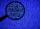A line of print on a computer contains 64 characters (letters, spacers or other chars). Find how many characters there are in 7 lines.
7. Cars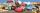Johnny has 370 cars. Peter twice less. How many cars have Peter?
8. A koala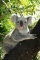A koala lives to be 14 years old in the wild. How much longer will a 2 year old koala probably live?
9. MultiplesFind all multiples of 10 that are larger than 136 and smaller than 214.
10. Salary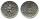Mr. Vesely got for work 874 CZK (Czech Republic Koruna). Mr. Jaros got twice less than Mr. Vesely. How much CZK got Mr. Jaros?
11. Number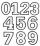Which number is 17 times larger than the number 6?
12. BreederSeven hens breeder has supplied 350 eggs. How long can deliver, if each of its chickens can withstand at least 5 eggs for week?
13. Family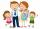Dad has two years more than mom. Mom has 5 times more than Katy. Katy has 2 times less than Jan. Jan is 10 years old. How old is everyone in the family? How old are all together?
14. Flour2 kg of flour costs 100 CZK. How much does it cost half a kilogram?
15. What isWhat is the value of the smaller of a pair of numbers for which their sum is 78 and their division quotients are 0.3?
16. Fridays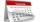Friday 13th is in 4 days. What day is today and what day it is?
17. Dividing by five and tenNumber 5040 divide by the number 5 and by number 10: a = 5040: 5 b = 5040: 10